Custom SearchCOMBINATIONS As indicated previously, a combination is the selection of a certain number of objects taken from a group of objects without regard to order. We use the symbol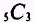to indicate that we have five objects taken three at a time, without regard to order. Using the letters A, B, C, D, and E to designate the five objects, we list the combinations as follows: ABC ABD ABE ACD ACE ADE BCD BCE BDE CDE We find 10 combinations of 5 objects are taken 3 at a time. The word combinations implies that order is not considered. EXAMPLE: Suppose we wish to know how many color combinations can be made from four different colored marbles if we use only three marbles at a time. The marbles are colored red, green, white, and yellow. SOLUTION: We let the first letter in each word indicate the color; then we list the possible combinations as follows: RGW RGY RWY GWY If we rearrange the groups, for example RGW, to form GWR or RWG, we still have the same color combination within each group; therefore, order is not important. The previous examples are relatively easy to understand; but suppose we have 20 boys and wish to know how many different basketball teams we could form, one at a time, from these boys. Our listing would be quite lengthy, and we would have a difficult task to determine we had all of the possible combinations. In fact, we would have to list over 15,000 combinations. This indicates the need for a formula. The general formula for possible combinations of r objects from a group of n objects is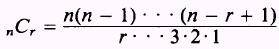The denominator may be written asand if we multiply both numerator and denominator bywhich is (n-r)! we have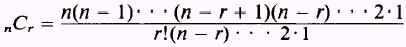The numerator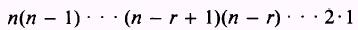isTherefore,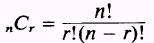This formula is read: The number of combinations of n objects taken r at a time is equal to n factorial divided by r factorial times n minus r factorial. EXAMPLE: In the previous problem where 20 boys were available, how many different basketball teams could be formed? SOLUTION: If the choice of which boy played center, guard, or forward is not considered, we find the number of combinations of 20 boys taken 5 at a time by writingwhere n=20 and r=5 Then, by substitution, we have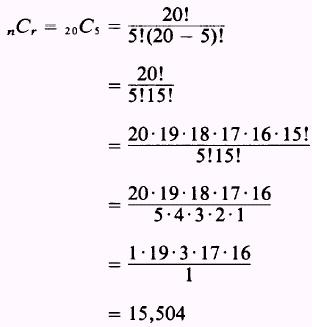EXAMPLE: A man has, in his pocket, a silver dollar, a halfdollar, a quarter, a dime, a nickel, and a penny. If he reaches into his pocket and pulls out three coins, how many different sums may he have? SOLUTION: The order is not important; therefore, the number of combinations of coins possible is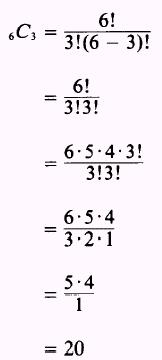EXAMPLE: Find the value of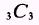SOLUTION: We use the formula given and find that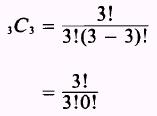This seems to violate the rule, division by zero is not allowed, but we define 0! to equal 1. Then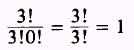which is obvious if we list the combinations of three things taken three at a time. PRACTICE PROBLEMS: Find the value of problems 1 through 6 and solve problems 7, 8, and 9.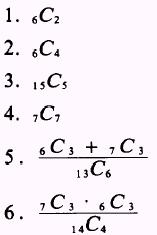7. We want to paint three rooms in a house, each a different color, and we may choose from seven different colors of paint. How many color combinations are possible for the three rooms? 8. If 20 boys go out for the football team, how many different teams may be formed, one at a time? 9. Two girls and their dates go to the drive-in, and each wants a different flavored ice cream cone. The drive-in has 24 flavors of ice cream. How many combinations of flavors may be chosen among the four of them if each one selects one flavor? ANSWERS: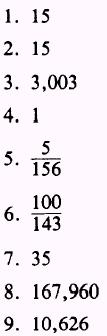Integrated Publishing, Inc. - A (SDVOSB) Service Disabled Veteran Owned Small Business